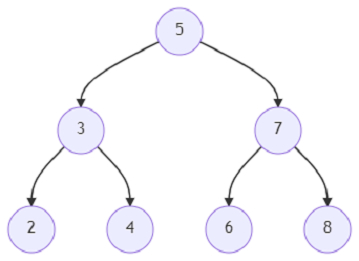# Program to find out the node in the right in a binary tree using Python

Suppose, we are provided a binary tree. We are also given a pointer to a node (named ‘u’) and we have to find the node situated just right of the provided node. The node situated to the given node's right must stay at the same level and the given node can either be a leaf node or an internal node.

So, if the input is likeand u = 6, then the output will be 8.

The node situated at the right of node 6 is node 8, so the value 8 is returned to us.

To solve this, we will follow these steps −

• if root is empty, then

• return null

• dq := a new deque

• insert root at the end of dq

• while dq is not empty, do

• dq_size := size of dq

• temp := a new list

• index := -1

• for each value in range 0 to dq_size, do

• node := delete last element from dq

• if left of node is not empty, then

• add left of node to the end of dq

• if right of node is not empty, then

• add right of node to the end of dq

• insert node at the end of temp

• if node is same as u, then

• index := size of temp - 1

• if index is same as size of temp - 1, then

• return null

• if index > -1, then

• return temp[index + 1]

• return null

Let us see the following implementation to get better understanding −

## Example

from queue import deque
class TreeNode:
def __init__(self, val=0, left=None, right=None):
self.val = val
self.left = left
self.right = right
def insert(temp,data):
que = []
que.append(temp)
while (len(que)):
temp = que
que.pop(0)
if (not temp.left):
if data is not None:
temp.left = TreeNode(data)
else:
temp.left = TreeNode(0)
break
else:
que.append(temp.left)
if (not temp.right):
if data is not None:
temp.right = TreeNode(data)
else:
temp.right = TreeNode(0)
break
else:
que.append(temp.right)
def make_tree(elements):
Tree = TreeNode(elements)
for element in elements[1:]:
insert(Tree, element)
return Tree
def search_node(root, element):
if (root == None):
return None
if (root.val == element):
return root
res1 = search_node(root.left, element)
if res1:
return res1
res2 = search_node(root.right, element)
return res2
def solve(root, u):
if not root:
return None
dq = deque()
dq.append(root)
while dq:
dq_size = len(dq)
temp = []
index = -1
for _ in range(dq_size):
node = dq.pop()
if node.left:
dq.appendleft(node.left)
if node.right:
dq.appendleft(node.right)
temp.append(node)
if node == u:
index = len(temp) - 1
if index == len(temp) - 1:
return None
if index > -1:
return temp[index + 1]
return None
root = make_tree([5, 3, 7, 2, 4, 6, 8])
u = search_node(root,6)
ret = solve(root, u)
print(ret.val)

## Input

root = make_tree([5, 3, 7, 2, 4, 6, 8])
u = search_node(root,6)

## Output

8